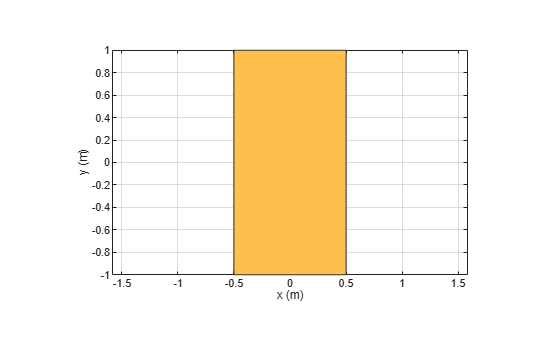# removeHoles

Remove holes from shape

Since R2020b

## Syntax

``s = removeHoles(shapeobject,holetol)``

## Description

example

````s = removeHoles(shapeobject,holetol)` removes holes with area less than the provided tolerance from the shape and returns a polygon object.```

## Examples

collapse all

Create two rectangular shapes with different dimensions.

```rect1 = antenna.Rectangle; rect2 = antenna.Rectangle(Length=1e-7, Width=0.5);```

Carve out rectangle2 from rectangle1 and view the resultant shape.

```rect3 = rect1 - rect2; show(rect3)```Remove holes from the resultant rectangle.

```rect4 = removeHoles(rect3,1e-6); show(rect4)```## Input Arguments

collapse all

2-D shape with sliver outlier, specified as either:

• Object created using `shapes` function.

• `shape.Rectangle`, `shape.Circle`, `shape.Ellipse`, or `shape.Polygon` object.

Data Types: `function`

Hole tolerance, specified as a nonnegative scalar.

Data Types: `double`

## Version History

Introduced in R2020b